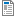National Curriculum

# Year 3 Maths Plans

We provide Hamilton Year 3 maths both as weekly plans (below) and as short blocks. We will eventually be phasing out the plans, as we believe our short blocks offer you all of the same advantages and more. Find out more about the advantages of Hamilton's short blocks.

Supporting documents for set
• Week
• Title
1
+ Details
Place value and money

Place two and three-digit numbers on a line; Order and compare three-digit numbers; Understand place value in three-digit numbers; Understand and use place value with moneyAutumn Week 1 Plan
2
+ Details

Know number bonds to 20 and use them in addition and subtraction; Use = to represent equality; Add 1-digit to 2-digit numbers; Subtract 1-digit from 2-digit numbers. Mental addition and subtraction using place value, partitioning and counting back. Includes bar model examples.Autumn Week 2 Plan
3
+ Details

Mental addition of pairs of two-digit numbers by partitioning; Mental subtraction of pairs of two-digit numbers by counting up; Find change from £1. Includes bar model examples.

4
+ Details
Shape and symmetry

Recognise lines of symmetry, complete symmetrical drawings; Describe, name and sort 2D shapes, Describe, name and sort 3D shapes, learn and use correct vocabulary.

5
+ Details
Multiplication and division

Use mental multiplication to double 2-digit numbers. Halve small even numbers. Know × and ÷ facts for 2, 5 and 10 times tables. Understand that multiplication is commutative. Recognise multiples of 2, 5 and 10. Includes bar model examples.

6
+ Details

Understand and use place value to add and subtract; Use place value in money to add and subtract; Add/subtract 1, 10 and 100 to/from any 3-digit number.

7
+ Details

Use place value to add and subtract 1s, 10s, 100s to/from 2 and 3-digit numbers; Mental addition and subtraction using near multiples of 10 from 3-digit numbers. Includes bar model examples.

8
+ Details

Know pairs of multiples of 5 totalling 100; Know pairs of 2-digit numbers totalling 100; Subtract numbers on either side of 100 by counting up

9
+ Details
Time and data

Tell the time to five minutes using analogue, digital and Roman numeral clocks; Understand and use am and pm times appropriately; Collect data and display using bar charts and pictograms

10
+ Details
Multiplication and division

Mental multiplication and division: Know multiplication facts for the 3 and 4 times tables up to the 12th multiple, derive corresponding division facts; Divide by 2, 3, 4, 5 and 10, including giving remainders.

11
+ Details
Fractions

Understand concept of a fraction of a shape and quantity; Find fractions of a quantity: ½, ⅓, ¼, ¾, ⅔. Includes bar model examples.